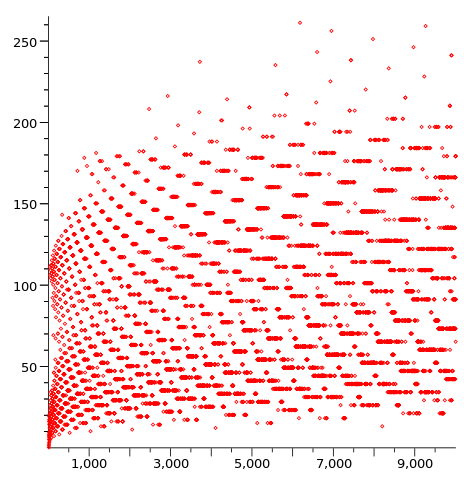# Collapsing from Collatz (300 followers problem!)

Calculus Level 4The Collatz conjecture (CC) is a well-known unsolved problem in mathematics, first proposed in 1937 by Lothar Collatz. Because of its overwhelming simplicity, the conjecture has been attacked many times - never successfully. The great Paul Erdős himself admitted: "Mathematics may not be ready for such problems."

Consider the function

$f(n) = \begin{cases} n/2 & \text{if } n \text{ is even} \\ 3n+1 &\text{if } n \text{ is odd} \end{cases}$

The conjecture states that all natural numbers when subjected to iteration of $f$ eventually end up at $1$.

Let the (minimum) number of iterations of $f$ taken by $n$ be $\ell(n)$. For example,

$5 \rightarrow 16 \rightarrow 8 \rightarrow 4 \rightarrow 2 \rightarrow 1$

Hence, $\ell(5) = 5$.

If $n$ never reaches $1$, then $\ell(n) = \infty$, with the interpretation that $\frac{1}{\ell(n)} = 0$.

What can be said about the convergence of the following series?

$\sum_{n=2}^{\infty} \frac{1}{\ell(n)}$

###### Image Credit: Wikimedia Cirne
×

Problem Loading...

Note Loading...

Set Loading...